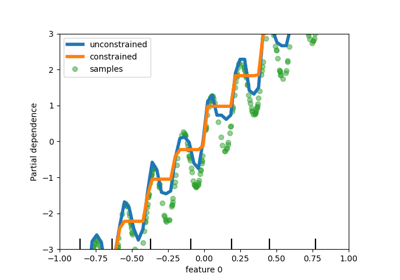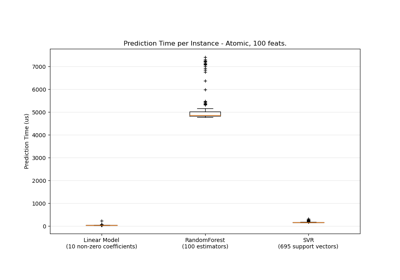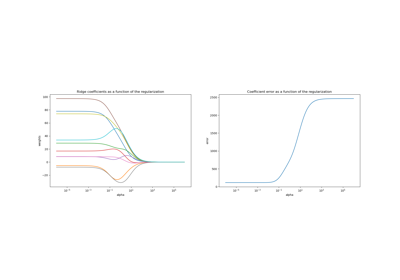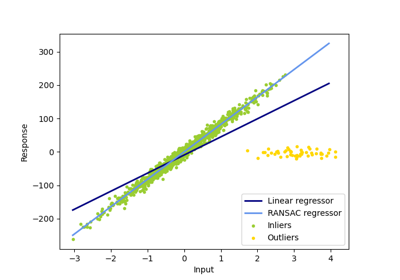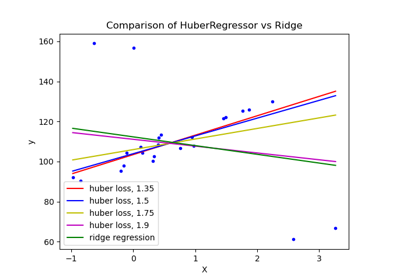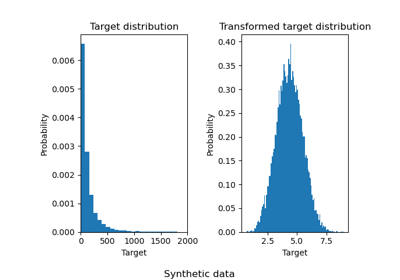# sklearn.datasets.make_regression¶

sklearn.datasets.make_regression(n_samples=100, n_features=100, *, n_informative=10, n_targets=1, bias=0.0, effective_rank=None, tail_strength=0.5, noise=0.0, shuffle=True, coef=False, random_state=None)[source]

Generate a random regression problem.

The input set can either be well conditioned (by default) or have a low rank-fat tail singular profile. See make_low_rank_matrix for more details.

The output is generated by applying a (potentially biased) random linear regression model with n_informative nonzero regressors to the previously generated input and some gaussian centered noise with some adjustable scale.

Read more in the User Guide.

Parameters
n_samplesint, optional (default=100)

The number of samples.

n_featuresint, optional (default=100)

The number of features.

n_informativeint, optional (default=10)

The number of informative features, i.e., the number of features used to build the linear model used to generate the output.

n_targetsint, optional (default=1)

The number of regression targets, i.e., the dimension of the y output vector associated with a sample. By default, the output is a scalar.

biasfloat, optional (default=0.0)

The bias term in the underlying linear model.

effective_rankint or None, optional (default=None)
if not None:

The approximate number of singular vectors required to explain most of the input data by linear combinations. Using this kind of singular spectrum in the input allows the generator to reproduce the correlations often observed in practice.

if None:

The input set is well conditioned, centered and gaussian with unit variance.

tail_strengthfloat between 0.0 and 1.0, optional (default=0.5)

The relative importance of the fat noisy tail of the singular values profile if effective_rank is not None.

noisefloat, optional (default=0.0)

The standard deviation of the gaussian noise applied to the output.

shuffleboolean, optional (default=True)

Shuffle the samples and the features.

coefboolean, optional (default=False)

If True, the coefficients of the underlying linear model are returned.

random_stateint, RandomState instance, default=None

Determines random number generation for dataset creation. Pass an int for reproducible output across multiple function calls. See Glossary.

Returns
Xarray of shape [n_samples, n_features]

The input samples.

yarray of shape [n_samples] or [n_samples, n_targets]

The output values.

coefarray of shape [n_features] or [n_features, n_targets], optional

The coefficient of the underlying linear model. It is returned only if coef is True.

## Examples using sklearn.datasets.make_regression¶